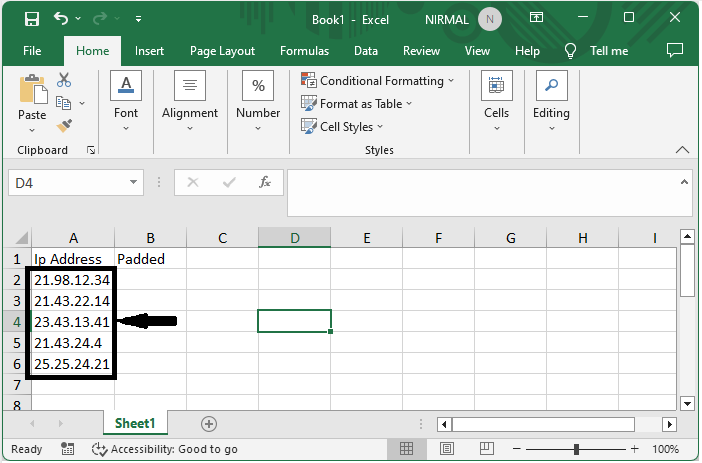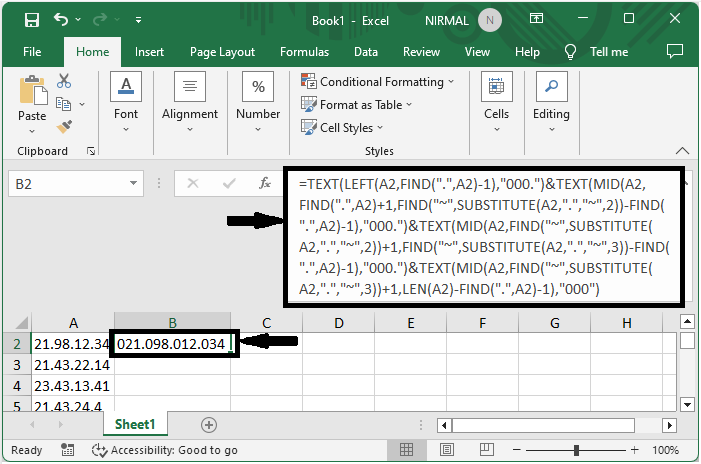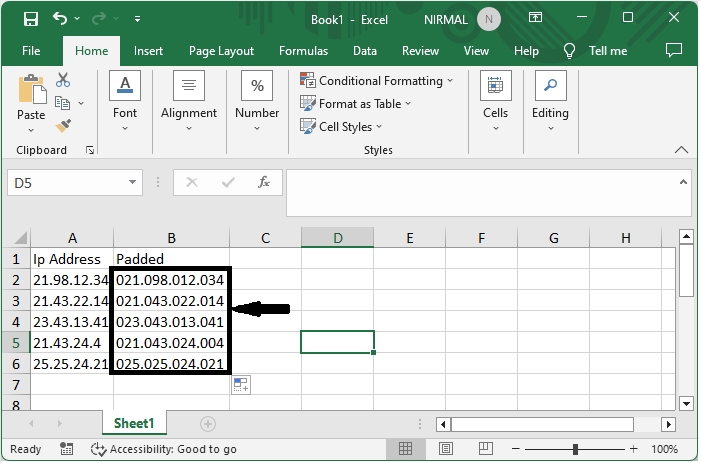IP addresses are incredibly important for identifying networked devices in the modern digital environment. Consistency in IP address format is crucial for all IP-related jobs, including network management, data analysis, and IP-related tasks. To maintain a uniform structure, it is a typical requirement to pad IP addresses with leading zeroes.

Here we will first get any one of the values using the formula, then use the autofill handle to complete the task. So let us see a simple process to know how you can pad an IP address with zero in Excel.

### Step 1

Consider an Excel sheet where you have a list of Internet Protocol addresses similar to the below image.First click on an empty cell, in this case cell B2, and enter the formula as

=TEXT(LEFT(A2,FIND(".",A2)-1),"000.")&TEXT(MID(A2,FIND(".",A2)+1,FIND

("~",SUBSTITUTE(A2,".","~",2))-FIND(".",A2)-1),"000.")&TEXT(MID(A2,FIND("~",SUBSTITUTE(A2,".","~",2))+1,FIND

("~",SUBSTITUTE(A2,".","~",3))-FIND(".",A2)-1),"000.")&TEXT(MID(A2,FIND("~",SUBSTITUTE(A2,".","~",3))+1,LEN(A2)-FIND(".",A2)-1),"000") and click enter to get the first value. In the formula, A1 is the address of the IP.

Empty Cell > Formula > Enter.### Step 2

Then drag down using the autofill handle to complete the task.This is how you can pad an IP address with a zero in Excel.

## Conclusion

In this tutorial, we have used a simple example to demonstrate how you can pad an IP address with zero in Excel to highlight a particular set of data.

Updated on: 06-Sep-2023

120 Views